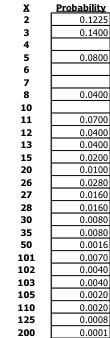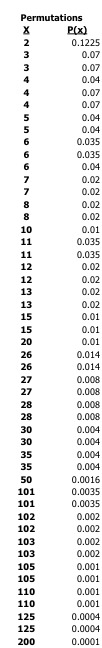# Return to Fred's Fun Factory (with 50 cents)

Alignments to Content Standards: S-CP.A.1 S-CP.A.2 S-CP.B.9

In order to play a popular “spinning wheel” game at Fred's Fun Factory Arcade, a player is required to pay a small, fixed amount of 25 cents each time he/she wants to make the wheel spin. When the wheel stops, the player is awarded tickets based on where the wheel stops -- and these tickets are then redeemable for prizes at a redemption center within the arcade.

This particular game has no skill component; each spin of the wheel is a random event, and the results from each spin of the wheel are independent of the results of previous spins.

The wheel awards tickets with the following probabilities:

 1 ticket 35% 2 tickets 20% 3 tickets 20% 5 tickets 10% 10 tickets 10% 25 tickets 4% 100 tickets 1%

A young girl is given 2 quarters so that she can play the game two times. Let $X$ be the number of tickets she wins based on two spins. There are 26 possible values for $X$ that the young girl can obtain in this case, and those values are listed to the right.

Some values of $X$ are more common than others. For example, winning only 2 tickets in two spins is a somewhat common occurrence with probability 0.1225 as it means the player earns 1 ticket on the first spin and 1 ticket on the second spin. Similarly, winning 200 tickets in two spins is a somewhat rare occurrence with probability 0.0001 as it means the player earns 100 tickets on the first spin and 100 tickets on the second spin. A full list of the possible values of $X$ and the corresponding probabilities for almost every value of $X$ is shown at right.

1. Four probability values are deliberately hidden. Determine the 4 missing probability values in the distribution. (Hint: since all values of $X$ are listed, and since the probabilities that are shown add up to 0.66, the 4 hidden probabilities you are computing should add up to very specific value.)
2. Which value of $X$ is most common?
3. The young girl considers it a "good day" with the game if she wins more than 100 tickets based on 2 spins. What is the probability that she will have a "good day" based on that definition?## IM Commentary

The task is intended to address standards regarding sample space, independence, probability distributions and permutations/combinations. Students might be inclined to use skills discussed in 7.SD.8. Some key components of this task that are intended to challenge some common misconceptions about probability distributions are as follows:

• Each possible value of $X$ is NOT equally likely.
• The most common $X$ value is NOT necessarily the result of the most common spin outcome occurring twice, i.e., just because a value of 1 is the most common spin value, that doesn't mean that the $X$ result of getting two 1's in two spins is the most common $X$ value.
• While there is only one outcome for certain $X$ values (e.g., 2, 10, 20, 50, 200), there are often 2 or even 3 outcomes that will generate other $X$ values.
• "Doubles" are not necessarily the only outcome that will generate certain $X$ values which are in fact double a possible spin value (e.g., while $X = 4$ can be obtained by 2 + 2 ["double 2's"], it can also be obtained by other outcomes).

Students should be aware that since the entire sample space is provided, the sum of all probabilities should be 1. This may help with verifying calculations in question #1, or perhaps a student will only compute 3 of the 4 unknown values in that question using permutation/independence logic and then compute the 4th value via subtraction.

Full detail of calculations is included for reference, but this is not required in order for students to complete this task.

Note: the information in the table has appeared in a companion exercise (Fred’s Fun Factory). A picture of a wheel fitting these parameters is included in that exercise.

## Solution

1. $$P(X = 4) = 0.18$$

$X = 4$ can be obtained by ordered spins of "1 then 3" or "2 then 2" or "3 then 1." Since these 3 outcomes are disjoint, the sum of the respective probabilities will yield the correct answer. Since each spin is independent, the probability of any outcome can be computed as the product of the probability of the first spin's value multiplied by the probability of the second spin's value.

Therefore, $P(1 \text{ then } 3) = .35 \cdot .2 = .07$; $P(2 \text{ then } 2) = .2 \cdot .2 = .04$; $P(3 \text{ then } 1) = .2 \cdot .35 = .07$.

The sum of these 3 probabilities is 0.18.

Note: students might use other methods such as a tree diagram approach to solve these questions. Hopefully, if that approach is taken, students will be economical in developing trees such that they do not include spin values that are greater than the $X$ value of interest.

$$P(X = 6) = 0.11$$

Using a similar approach as above: $P(1 \text{ then } 5) = .35 \cdot .10 = .035$; $P(3 \text{ then } 3) = .2 \cdot .2 = .04$; $P(5 \text{ then } 1) = .10 \cdot .35 = .035$.

The sum of these 3 probabilities is 0.11.

$$P(X = 7) = 0.04$$

Using a similar approach as above: $P(2 \text{ then } 5) = .20 \cdot .10 = .02$; $P(5 \text{ then } 2) = .10 \cdot .20 = .02$.

The sum of these 2 probabilities is 0.04.

$$P(X = 10) = 0.01$$

$P(5 \text{ then } 5) = .10 \cdot .10 = .01$. This is the only permutation for $X = 10$.

Note: The 4 "$X$" probabilities will add up to .34 since the remaining probability distribution values shown in the task add up to .66. Thus a student might compute one of the 4 probabilities using subtraction (e.g., .34 – "3 other $X$ probabilities" = 4th $X$ probability, and so on) based on the assumption that the 3 other calculations are correct.

2. $X = 4$ has the highest probability at 0.18 (Students will need to compute values correctly in question #1 to obtain this answer.)
3. There are 7 values of $X$ that are greater than 100.  The sum of the corresponding 7 probabilities is 0.0199. (Students do NOT need to compute values correctly in question (a) to obtain this answer.)